## Abstract

Plasma cells (PCs) can be grouped into subsets based on the isotype of the antibody they secrete, their phenotype including maturity and their survival niche in the body. In this study we examined differential gene expression in several of these subsets. We sorted PCs from lamina propria (LP), bone marrow (BM) and spleens (SPL) collected from three wild type C57Bl/6 mice from which we prepared RNA to analyse by microarray. We further split BM and SPL samples into B220+ and B220- subsets while all LP PCs used were B220-. We also sorted folicular B cells from these mice for a total of 3 samples from each of 6 groups for a total of 18 samples. The following report includes normalization data, exploratory principle component analysis and differentially expressed gene analysis for two experimental conditions. These were examination of the effect of organ of residence of mature, B220- PCs and examination of the effect of maturity in both BM and SPL PCs comparing B220- and B220+ subsets.

## R packages

These R/bioconductor packages were used to complete the analysis of these Affy1.0 arrays.

``````library(oligo)
library(affycoretools)
library(pd.mogene.1.0.st.v1)
library(mogene10sttranscriptcluster.db)
library(RColorBrewer)
library(gplots)
library(ggplot2)
library(genefilter)
library(limma)
library(annotate)
library(reshape2)
library(Biobase)``````

This report was compiled in Rmarkdown using these packages:

``````library(rmarkdown)
library(knitr)
library(devtools)``````

## Experimental set up and Quality Control

First read in a textfile containing the experimental design and assign sample names

``````targets <- read.delim("allmanArray_4821_studyDesignFile.txt", sep="\t", stringsAsFactors = FALSE)
sampleLabels <- as.character(paste(targets\$Tissue, targets\$cellType, targets\$B220, targets\$replicate, sep="."))
myGroups <- factor(paste(targets\$Tissue, targets\$cellType, targets\$B220, sep="."))``````

Now read in and normalize the raw data from the CEL files

``````rawData <- read.celfiles(list.celfiles())
normData <- oligo::rma(rawData, target="core")``````

#### Figure 1: Histogram of signal intensity across each array before (A) and after normalization (B)

``````cols <- topo.colors (n=18, alpha=1)
hist(rawData, xlab = "log2 expression", main = "A: Non-normalized data - histograms", col=cols)``````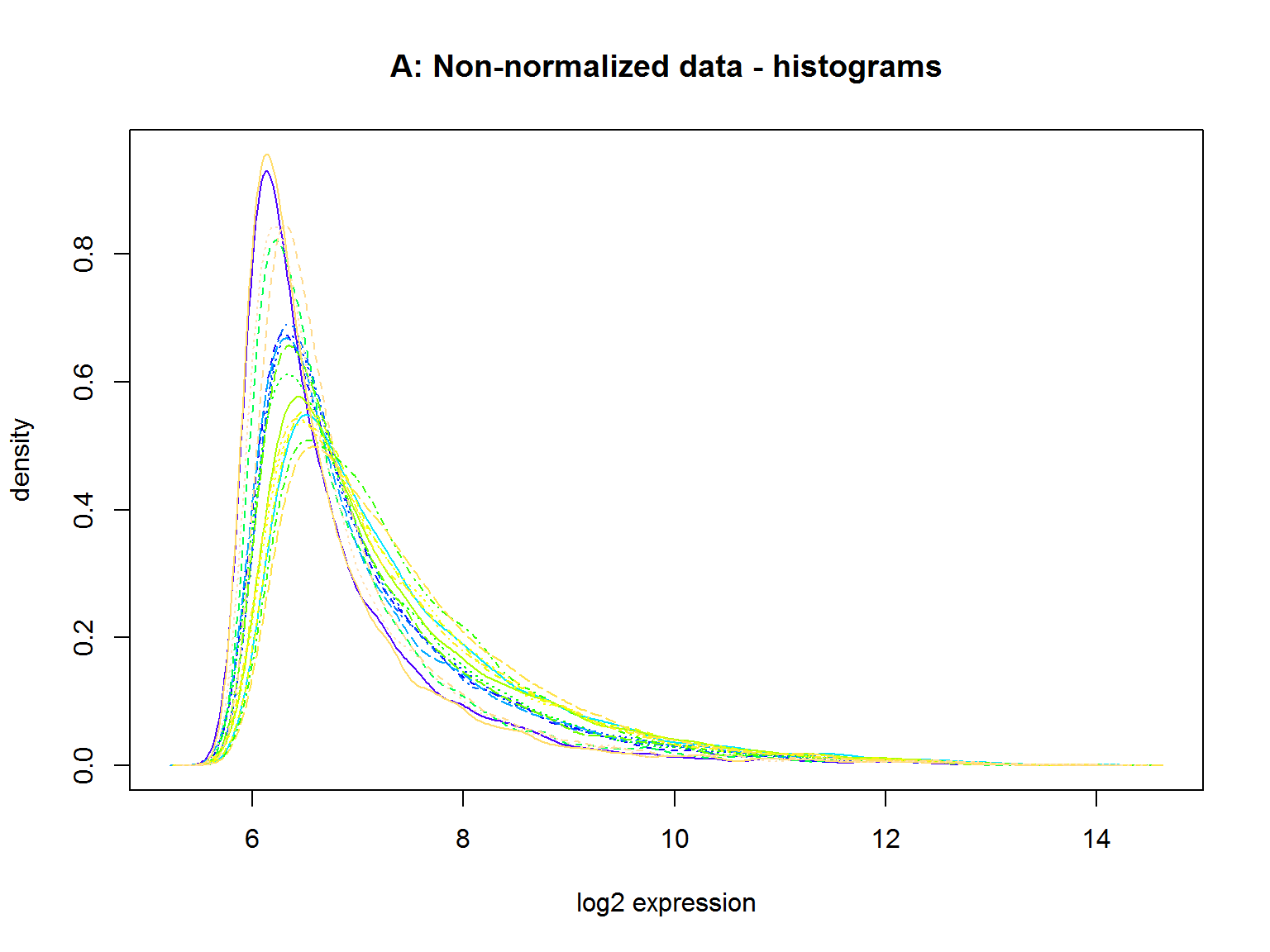``hist(normData, xlab = "log2 expression", main = "B: Normalized data - histograms", col=cols)``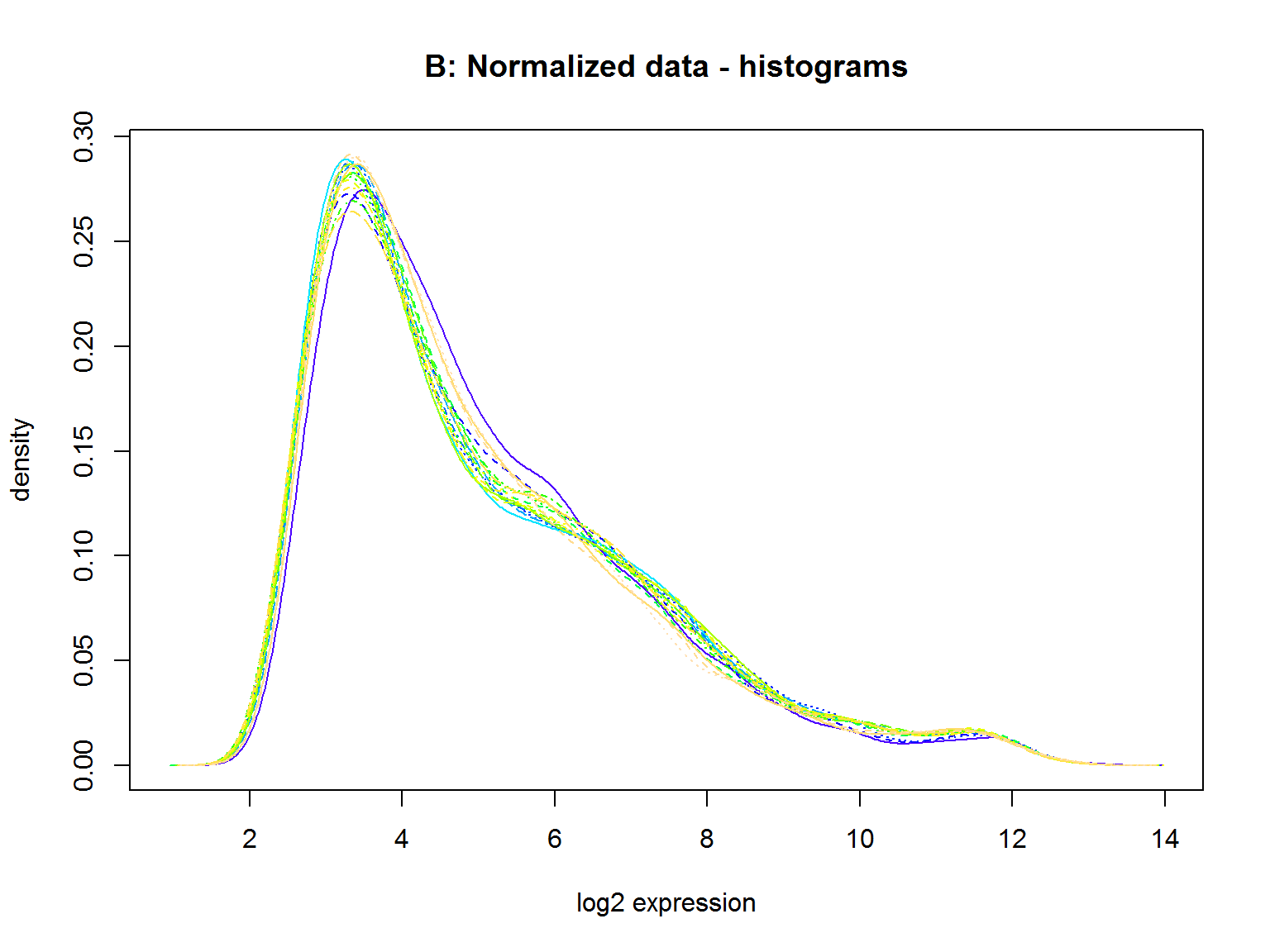#### Figure 2: Box plot representation of raw (A) and normalized (B) data

``````boxplot(rawData, names = sampleLabels, ylab = "non-normalized log2 expression",
main = "A: Non-normalized data - boxplots", col=cols)``````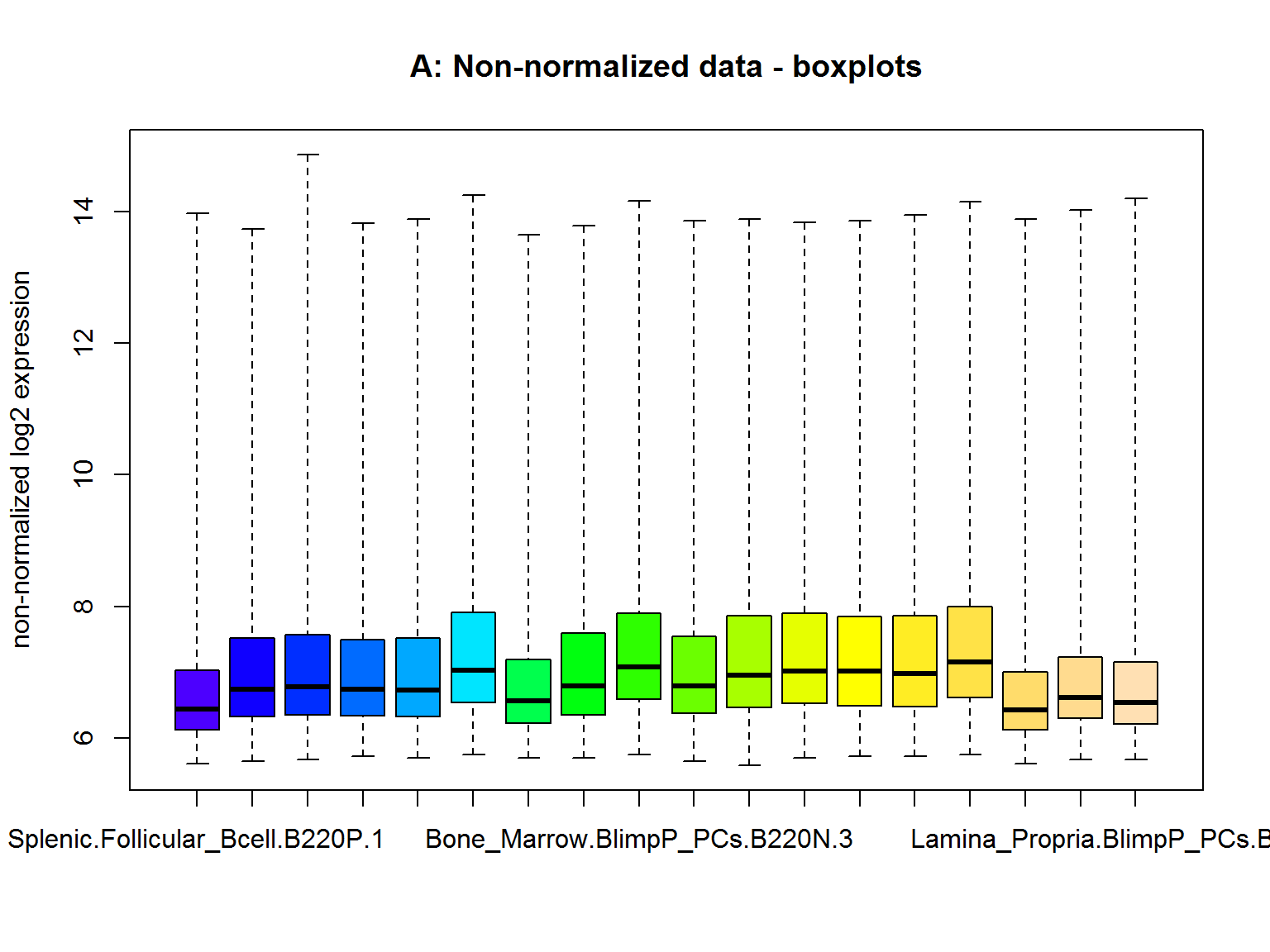``````boxplot(normData, names = sampleLabels, ylab = "normalized log2 expression",
main = "B: Normalized data - boxplots", col=cols)``````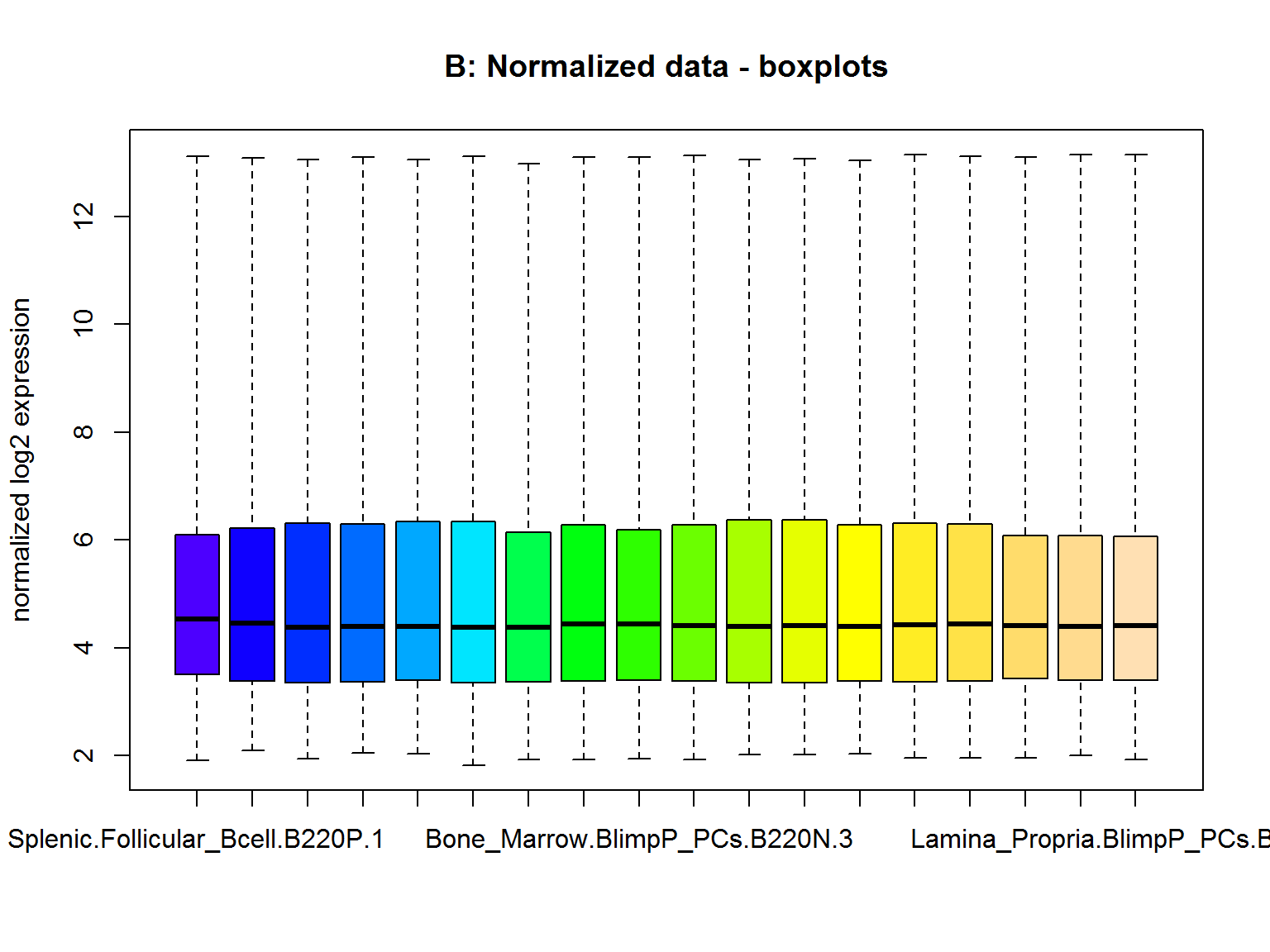Now filter the data to remove control probes and duplicates keeping probes with max signal.
Also remove probes not detected over background or with low varience over all samples.
Create a datamatrix containing the normalized filtered data.

``````normData.main <- getMainProbes(normData)
normData.main.matrix <- exprs(normData.main)
annotation(normData) <- "mogene10sttranscriptcluster.db"
filtered_geneList <- nsFilter(normData, var.func=IQR, var.filter=TRUE, var.cutoff=0.5, filterByQuantile=TRUE, feature.exclude="^AFFX")
filtered.eset <- filtered_geneList\$eset
filtered.matrix <- as.matrix(filtered.eset)
probeList <- rownames(filtered.matrix)``````

Discussion 1: The raw data looks pretty good and the normalization seems to be satisfactory. After normalization and filtering there are 10431 genes in the data set.

## Data Exploration

Now hierarchical clustering and principle component analysis of the data

#### Figure 3. Hierarchical clustering of plasma cell subsets.

``````distance <- dist(t(filtered.matrix),method="euclidian")
clusters <- hclust(distance, method = "average")
plot(clusters, label = sampleLabels, hang = -1, main="Plasma cell Subsets")``````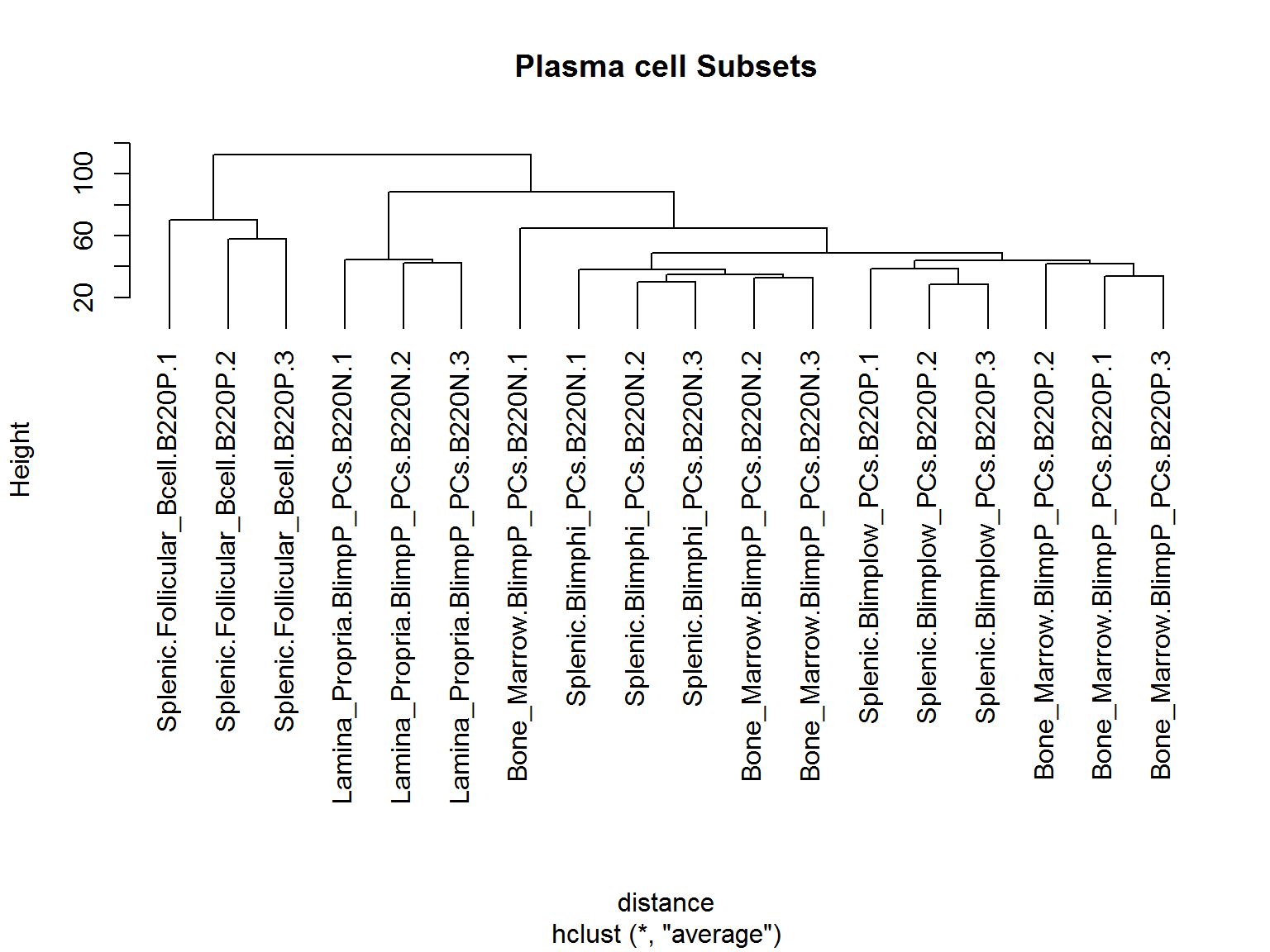Discussion 2a: The sample Bone_Marrow.BlimpP.B220N.1 is not clustering with its biological replicates. Furthermore, it appears to be less similar to its replicates than all of the other PC samples except LP PCs.

#### Figure 4. Principle component analysis showing that the bulk of the varience is accounted for in pc1 and pc2

``````pca.res <- prcomp(t(filtered.matrix), scale.=F, retx=T)
ls(pca.res)
summary(pca.res)
plot(pca.res, las=1)``````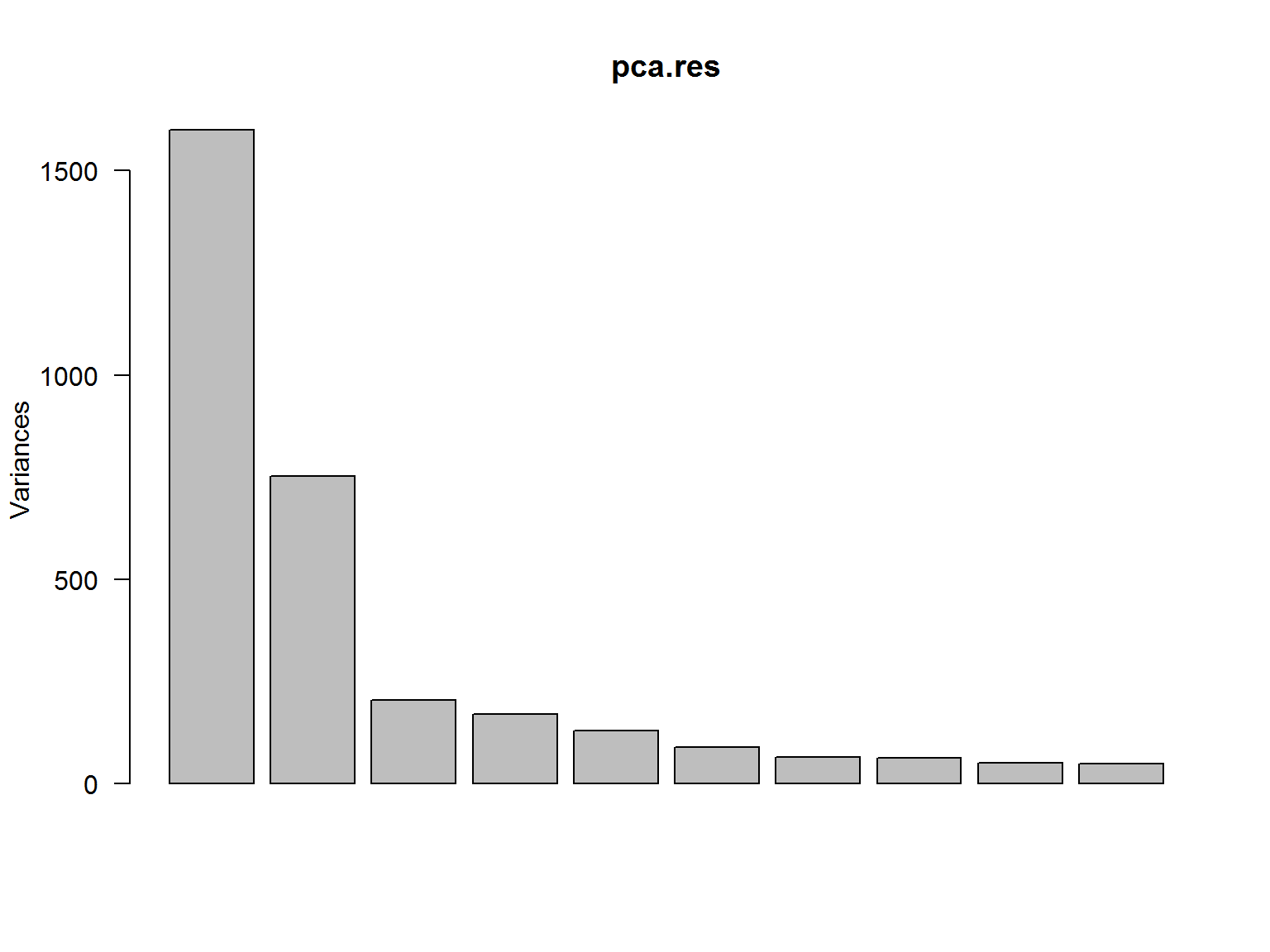numerical contribution of PCs to total variance

``````pc.var<-pca.res\$sdev^2
pc.per<-round(pc.var/sum(pc.var)*100, 1)
pc.per``````
``````##   47.4 22.3  6.0  5.0  3.8  2.6  1.9  1.8  1.5  1.4  1.3  1.1  1.0  0.8
##   0.7  0.7  0.6  0.0``````

#### Figure 5. Contibution of samples to PC1 and PC2.

``````data.frame <- as.data.frame(pca.res\$x)
ggplot(data.frame, aes(x=PC1, y=PC2, colour=factor(myGroups))) +
geom_point(size=5) +
theme(legend.position="right")``````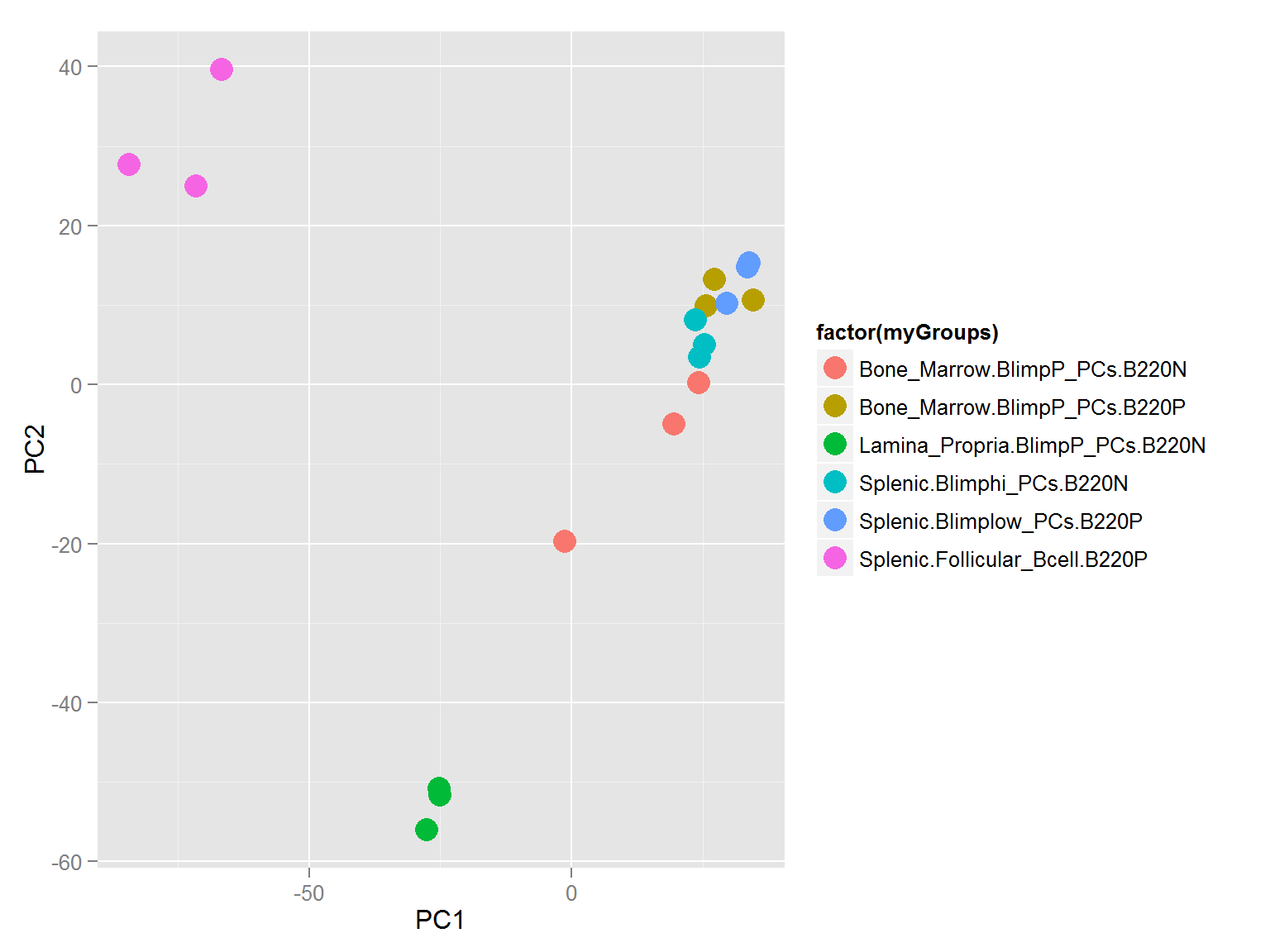#### Figure 6. Small multiples chart to examine the contribution of each experimental variable on each PC.

``````melted <- cbind(myGroups, melt(pca.res\$x[,1:9]))
ggplot(melted) +
geom_bar(aes(x=Var1, y=value, fill=myGroups), stat="identity") +
facet_wrap(~Var2)``````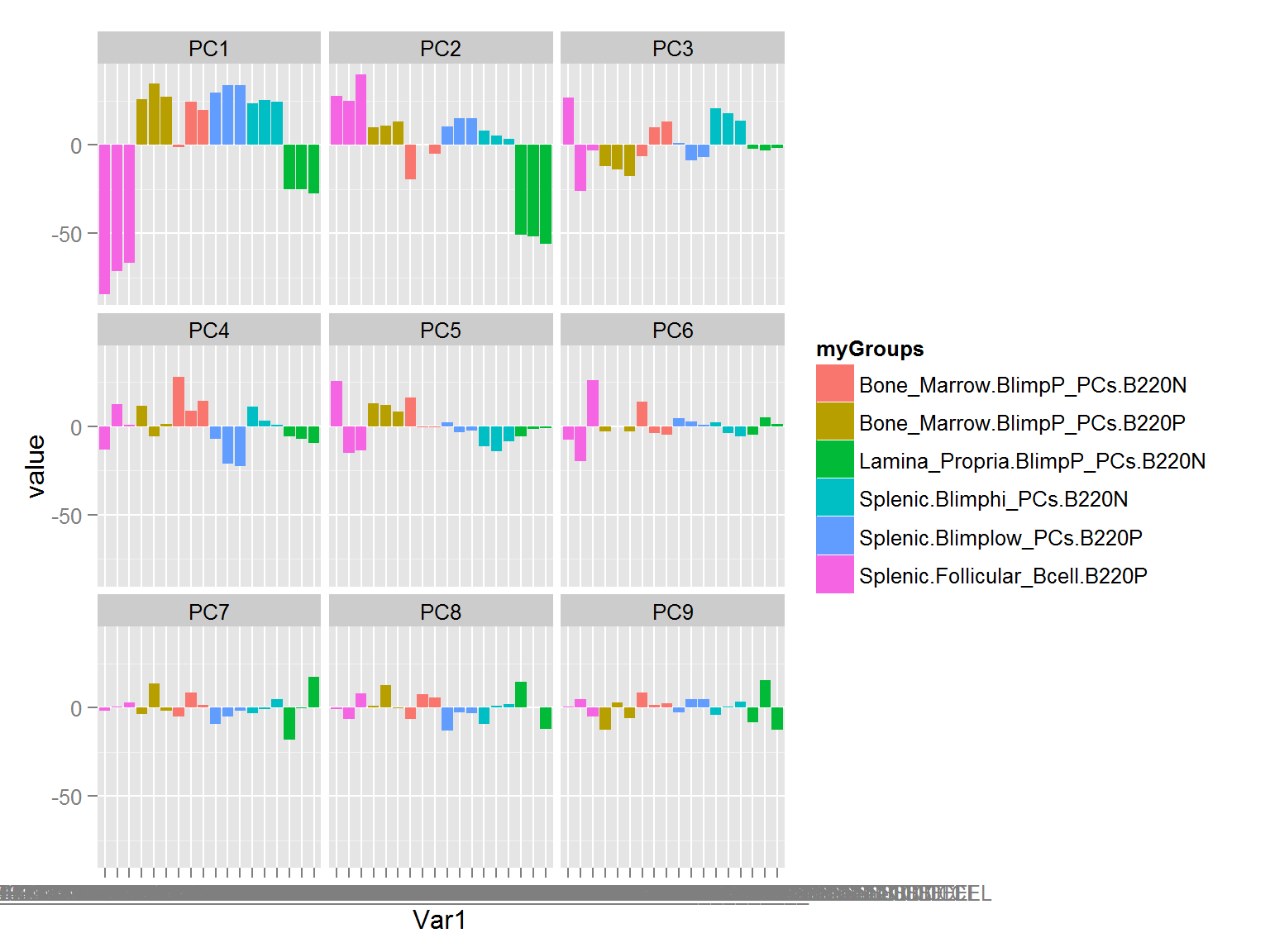Discussion 2b: Sample Bone_Marrow.BlimpP.B220N.1 which didn’t cluster well on the dendogram also seems to be wandering on the principle component analysis. We’ll keep an eye on it for future analysis. Looking at the PCA results we see that PC1 accounts for 47.4% of the variance and is driven primarily by the Splenic Folicular B cell group and to a lesser extent the Lamina propria plasma cell group. PC2 contributes 22.3% of the variance and appears to be driven completely by the Lamina propria PCs. Since PC1 and PC2 account for 69.7% of the total variance we will focus first on the differentially expressed genes in B220 negative plasma cells in the Lamina propria, Spleen and Bone Marrow.

## Differentially Expressed Genes 1: Lamina Propria PCs

Now set up the experimental design and think about the question to ask in this analysis.
The bulk of the variability in the PC groups was between Lamina propria and all other plasma cells so let’s look there first.

``````design <- model.matrix(~0+myGroups)
colnames(design) <- levels(myGroups)
design``````

Define the contrast matrix and look at differentially expressed genes.

``````fit <- lmFit(filtered.matrix, design)
fit\$genes\$Symbol <- getSYMBOL(probeList, "mogene10sttranscriptcluster.db")
fit\$genes\$Entrez <- getEG(probeList, "mogene10sttranscriptcluster.db")
contrast.matrix.LP <- makeContrasts(LPvBM = Lamina_Propria.BlimpP_PCs.B220N - Bone_Marrow.BlimpP_PCs.B220N,
LPvSP = Lamina_Propria.BlimpP_PCs.B220N - Splenic.Blimphi_PCs.B220N,
BMvSP = Bone_Marrow.BlimpP_PCs.B220N - Splenic.Blimphi_PCs.B220N,
levels=design)
fits <- contrasts.fit(fit, contrast.matrix.LP)
ebFit <- eBayes(fits)``````

Let’s take a look at the top 20 differentially expressed genes between LP and BM PCs.

``````myTopHits <- topTable(ebFit, adjust ="BH", coef=1, number=20, sort.by="logFC")
row.names(myTopHits) <- myTopHits[,1]
myTopHits <- myTopHits[,c(3,4,6,7)]
knitr::kable(myTopHits)``````
S100a8 -6.882960 9.003908 0.0000007 0.0000658
S100a9 -6.047951 8.896159 0.0000003 0.0000376
AY761184 5.853411 4.265125 0.0000000 0.0000000
Lgals2 5.768091 4.575247 0.0000000 0.0000000
Lypd8 5.133256 4.670593 0.0000000 0.0000000
Anpep 5.084076 4.621503 0.0000000 0.0000006
Lyz1 4.888409 5.711908 0.0000000 0.0000001
Hspa1a 4.777929 6.510416 0.0000000 0.0000000
Gpx2 4.709698 4.339741 0.0000000 0.0000000
Dusp4 4.579407 5.535097 0.0000000 0.0000007
Camp -4.498698 7.474374 0.0000097 0.0004113
Tff2 4.453222 4.718739 0.0000000 0.0000021
Ccl3 4.425484 4.975895 0.0000002 0.0000319
Ngp -4.345148 6.320623 0.0000225 0.0006812
Cxcl10 4.314690 6.032995 0.0000000 0.0000000
C1qc 4.237419 6.188722 0.0000339 0.0008758
Lcn2 -4.065409 6.318030 0.0001453 0.0022935
C1qb 4.049342 5.825150 0.0000014 0.0001106
Clca4 3.869933 4.562927 0.0000000 0.0000000
Atf3 3.817459 4.413907 0.0000000 0.0000004

…and now between LP and Spleen…

``````myTopHits <- topTable(ebFit, adjust ="BH", coef=2, number=20, sort.by="logFC")
row.names(myTopHits) <- myTopHits[,1]
myTopHits <- myTopHits[,c(3,4,6,7)]
knitr::kable(myTopHits)``````
Lgals2 5.819339 4.575247 0.0000000 0.0000000
AY761184 5.785074 4.265125 0.0000000 0.0000000
Hspa1a 5.574776 6.510416 0.0000000 0.0000000
Lypd8 5.346594 4.670593 0.0000000 0.0000000
Lyz1 5.324838 5.711908 0.0000000 0.0000000
Anpep 5.238278 4.621503 0.0000000 0.0000003
Ccl3 4.777648 4.975895 0.0000001 0.0000071
Gpx2 4.673459 4.339741 0.0000000 0.0000000
Tff2 4.459495 4.718739 0.0000000 0.0000013
Chst1 -4.341668 7.997142 0.0000000 0.0000005
Ms4a1 -4.160032 7.414913 0.0000000 0.0000000
Cxcl10 4.114892 6.032995 0.0000000 0.0000000
Atf3 4.061560 4.413907 0.0000000 0.0000001
Dusp4 3.983158 5.535097 0.0000000 0.0000021
Ctrb1 3.914233 4.497992 0.0006153 0.0032075
C1qb 3.908739 5.825150 0.0000022 0.0000604
C1qc 3.903429 6.188722 0.0000841 0.0007283
Apoa1 3.898802 6.331444 0.0000000 0.0000000
Clca4 3.890256 4.562927 0.0000000 0.0000000
Il5ra -3.876412 5.721173 0.0000008 0.0000321

…and finally between BM and Spleen.

``````myTopHits <- topTable(ebFit, adjust ="BH", coef=3, number=20, sort.by="logFC")
row.names(myTopHits) <- myTopHits[,1]
myTopHits <- myTopHits[,c(3,4,6,7)]
knitr::kable(myTopHits)``````
Camp 4.226134 7.474374 0.0000204 0.0532411
Ngp 4.013620 6.320623 0.0000556 0.0558541
S100a8 3.884341 9.003908 0.0004968 0.1359324
S100a9 3.792425 8.896159 0.0000948 0.0660801
Lcn2 3.762065 6.318030 0.0003163 0.1218248
Retnlg 3.319330 6.947406 0.0021214 0.2377735
Mpo 3.247564 5.581281 0.0000897 0.0660801
Chil3 3.088146 5.320545 0.0025242 0.2377735
Ifitm6 2.548911 5.296799 0.0185243 0.4173375
Ltf 2.349185 5.245582 0.0118634 0.3693948
Elane 1.951168 4.927956 0.0066362 0.3204709
Alox5ap 1.926839 5.954010 0.0091774 0.3528797
Prtn3 1.893833 4.910396 0.0003387 0.1218248
Hp 1.892652 4.886293 0.0207325 0.4377741
Mmp8 1.775127 4.152329 0.0173082 0.4038974
Plekhf1 1.768204 5.429450 0.0000447 0.0558541
D730005E14Rik -1.759264 4.929671 0.0055348 0.2960695
Wfdc21 1.759028 5.406629 0.0067267 0.3218639
Erc1 1.752971 5.555207 0.0003350 0.1218248
Mt1 1.750117 9.325127 0.0040936 0.2772326

Then we’ll create a ven diagram showing differential gene expression and overlap between these groups.

#### Figure 7. Differentially expressed genes by subset

``````results <- decideTests(ebFit, method="global", adjust.method="BH", p.value=0.05, lfc=0.59)
#results <- decideTests(ebFit, method="global", adjust.method="BH", p.value=0.05)
vennDiagram(results, include="both")``````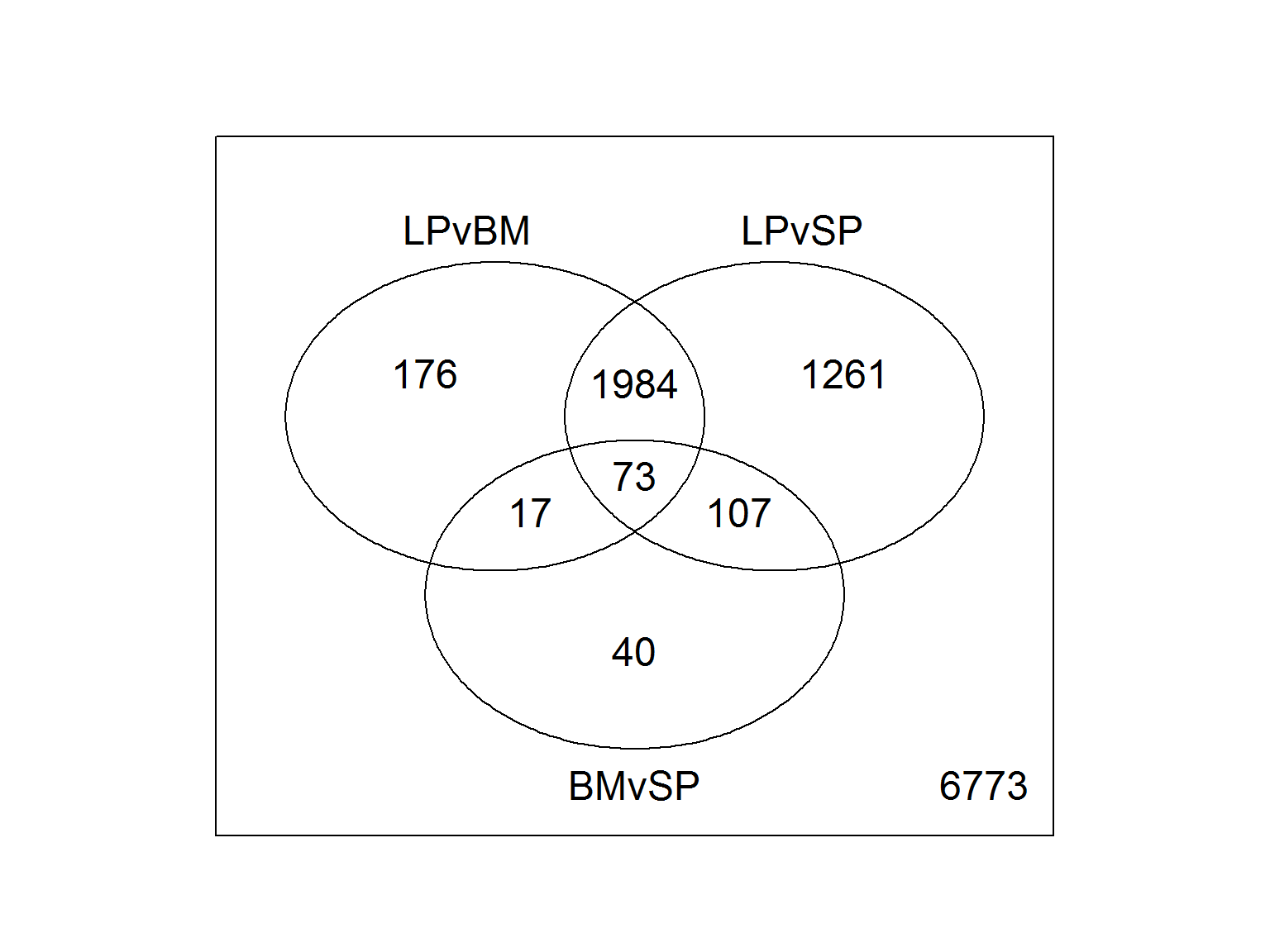Pull out and subset the differentially expressed genes.

``````diffProbes <- which(results[,1] !=0 | results[,2] !=0 | results[,3] !=0)
diffSymbols <- fit\$genes\$Symbol[results[,1] !=0 | results[,2] !=0 | results[,3] !=0]
diffEntrez <- fit\$genes\$Entrez[results[,1] !=0 | results[,2] !=0 | results[,3] !=0]
myEset.ALL <- new("ExpressionSet", exprs = filtered.matrix)
annotation(myEset.ALL) <- "mogene10sttranscriptcluster.db"
diffData <- filtered.eset[results[,1] !=0 | results[,2] !=0 | results[,3] !=0]
diffData <- exprs(diffData)``````

Subset the data to include B220N PCs from LP Spleen and BM and create a heat map of these genes
Let’s look at the heat map without averaging the replicates first to get a better idea of the contribution of B220N.1 from the BM that was previously discussed.

#### Figure 8. Heat map of Differentially expressed genes in B220 negative subsets

``````diffData.subset <- diffData[,c(7,8,9,13,14,15,16,17,18)]
hr <- hclust(as.dist(1-cor(t(diffData.subset), method="pearson")), method="complete") #cluster rows by pearson correlation
hc <- hclust(as.dist(1-cor(diffData.subset, method="spearman")), method="complete") #cluster columns by spearman correlation
mycl <- cutree(hr, k=4)
mycolhc <- rainbow(length(unique(mycl)), start=0.1, end=0.9)
mycolhc <- mycolhc[as.vector(mycl)]
myheatcol <- greenred(75)
heatmap.2(diffData.subset, Rowv=as.dendrogram(hr), Colv=NA,
col=myheatcol, scale="row", labRow=NA, labCol=NA,
density.info="none", trace="none", RowSideColors=mycolhc,
cexRow=1, cexCol=1, margins=c(8,30))``````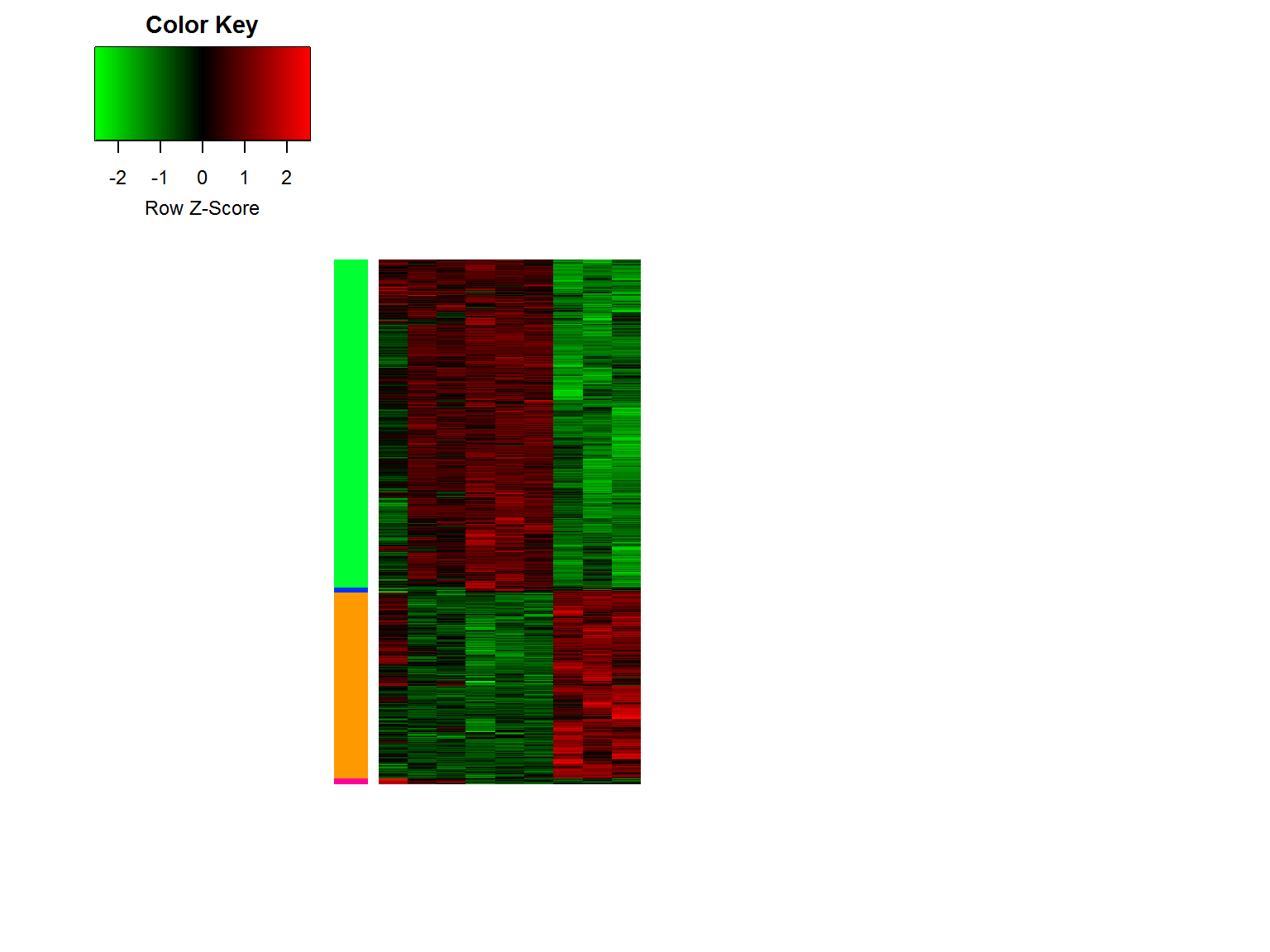Now average the biological replicates for a more clear heatmap

#### Figure 9. Heat map of Differentially expressed genes in B220 negative subsets

``````colnames(diffData) <- myGroups
rownames(diffData) <- diffSymbols
diffData.AVG <- avearrays(diffData)
diffData.AVG.subset <- diffData.AVG[,c(3,5,6)]
heatmap.2(diffData.AVG.subset, Rowv=as.dendrogram(hr), Colv=NA,
col=myheatcol, scale="row", labRow=NA, labCol=colnames(diffData.AVG.subset),
density.info="none", trace="none", RowSideColors=mycolhc,
cexRow=1, cexCol=1, margins=c(15,30))``````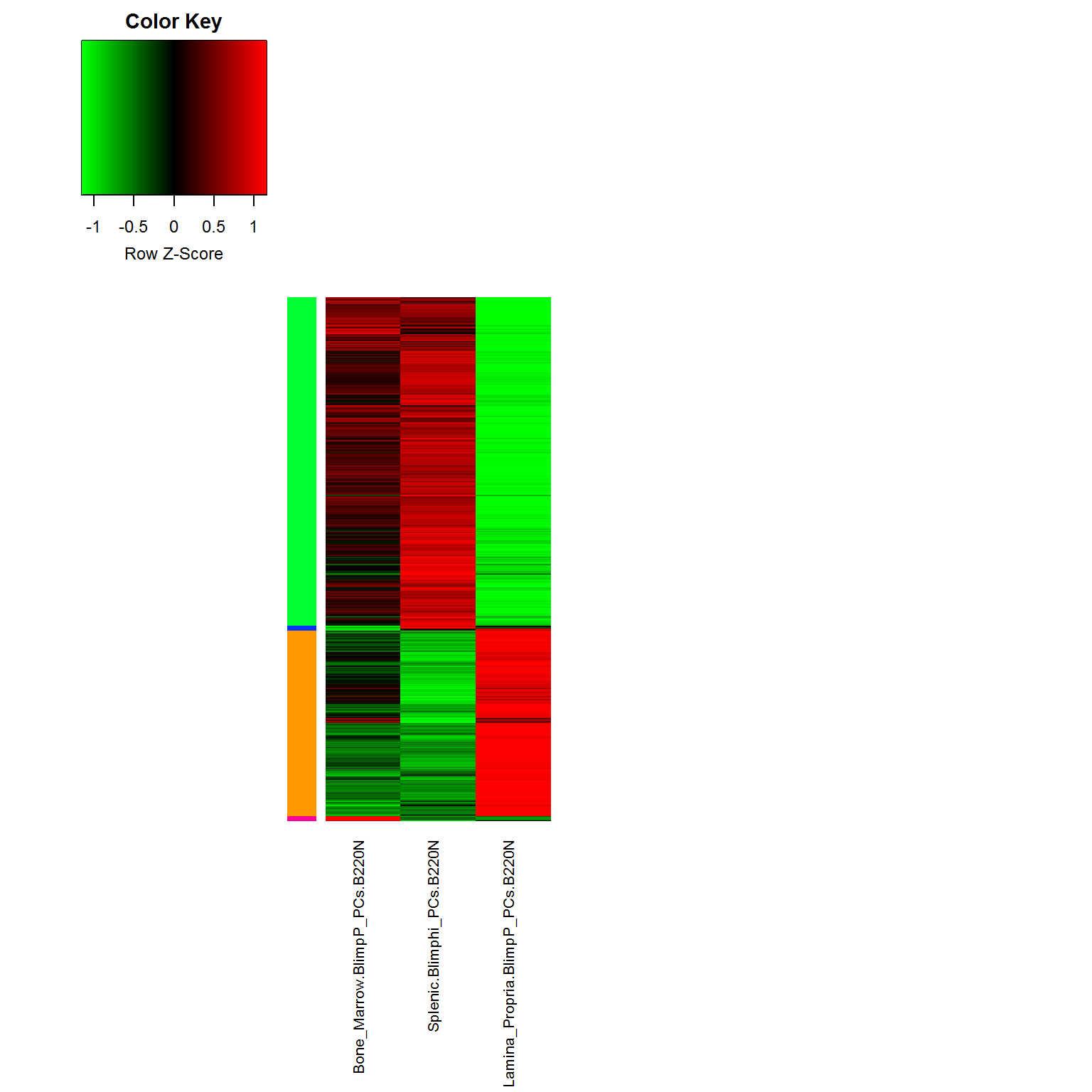Take a look at the genes in each cluster and think about downstream enrichment analyses.

``````hr <- hclust(as.dist(1-cor(t(diffData.AVG.subset), method="pearson")), method="complete") #cluster rows by pearson correlation
hc <- hclust(as.dist(1-cor(diffData.AVG.subset, method="spearman")), method="complete") #cluster columns by spearman correlation

clid <- c(1)
ysub <- diffData.subset[names(mycl[mycl%in%clid]),]
hrsub <- hclust(as.dist(1-cor(t(ysub), method="pearson")), method="complete")
clusterIDs <- data.frame(Labels=rev(hrsub\$labels[hrsub\$order]))
clusterIDs_1 <- as.vector(t(clusterIDs))

clid <- c(2)
ysub <- diffData.subset[names(mycl[mycl%in%clid]),]
hrsub <- hclust(as.dist(1-cor(t(ysub), method="pearson")), method="complete")
clusterIDs <- data.frame(Labels=rev(hrsub\$labels[hrsub\$order]))
clusterIDs_2 <- as.vector(t(clusterIDs))

clid <- c(3)
ysub <- diffData.subset[names(mycl[mycl%in%clid]),]
hrsub <- hclust(as.dist(1-cor(t(ysub), method="pearson")), method="complete")
clusterIDs <- data.frame(Labels=rev(hrsub\$labels[hrsub\$order]))
clusterIDs_3 <- as.vector(t(clusterIDs))

clid <- c(4)
ysub <- diffData.subset[names(mycl[mycl%in%clid]),]
hrsub <- hclust(as.dist(1-cor(t(ysub), method="pearson")), method="complete")
clusterIDs <- data.frame(Labels=rev(hrsub\$labels[hrsub\$order]))
clusterIDs_4 <- as.vector(t(clusterIDs))``````

#### Differential genes in each cluster

ClusterID Phenotype number of genes
Cluster 1 up in LP 1296
Cluster 2 down in LP 2289
Cluster 3 up in BM 36
Cluster 4 up in SPL 37

## Differentially Expressed Genes 2: B220+ and B220- PCs

Now set up the experimental design and think about the question to ask in this analysis.
This time we’ll look at B220 positive verses negative in BM and Spleen

``````design <- model.matrix(~0+myGroups)
colnames(design) <- levels(myGroups)
design``````

Define the contrast matrix and look at differentially expressed genes.

``````fit <- lmFit(filtered.matrix, design)
fit\$genes\$Symbol <- getSYMBOL(probeList, "mogene10sttranscriptcluster.db")
fit\$genes\$Entrez <- getEG(probeList, "mogene10sttranscriptcluster.db")
contrast.matrix.B220 <- makeContrasts(BM = Bone_Marrow.BlimpP_PCs.B220N - Bone_Marrow.BlimpP_PCs.B220P,
SP = Splenic.Blimphi_PCs.B220N - Splenic.Blimplow_PCs.B220P,
BMvSP = Bone_Marrow.BlimpP_PCs.B220N - Splenic.Blimphi_PCs.B220N,
levels=design)
fits <- contrasts.fit(fit, contrast.matrix.B220)
ebFit <- eBayes(fits)``````

Let’s take a look at the top 20 differentially expressed genes between B220N vs B220P in the BM.

``````myTopHits <- topTable(ebFit, adjust ="BH", coef=1, number=20, sort.by="logFC")
row.names(myTopHits) <- myTopHits[,1]
myTopHits <- myTopHits[,c(3,4,6,7)]
knitr::kable(myTopHits)``````
Prr11 -2.600791 6.070410 0.0000205 0.0142506
2810417H13Rik -2.360435 5.825327 0.0009263 0.0671002
Ncapg -2.268771 5.656746 0.0000000 0.0003858
Samd9l -2.263077 5.956477 0.0000028 0.0086732
Top2a -2.249430 6.511040 0.0004025 0.0471228
Kif11 -2.172195 5.596486 0.0001021 0.0273212
Cdkn3 -2.155774 5.926671 0.0000237 0.0154548
Casc5 -2.127347 5.203823 0.0001146 0.0282446
Smc2 -2.105534 4.855630 0.0000691 0.0236995
Birc5 -2.056770 6.522428 0.0009937 0.0681471
Hmmr -2.038312 4.652314 0.0003026 0.0409255
Cenpe -2.024122 4.168182 0.0000315 0.0173086
Mki67 -2.000671 7.602688 0.0005751 0.0517115
Gm10796 -1.977119 4.931081 0.0010516 0.0689858
Xist -1.970199 6.190671 0.3492558 0.6277938
Marcks -1.968983 5.511916 0.0000907 0.0262802
Cenpk -1.916631 4.772934 0.0000344 0.0179515
Arhgap11a -1.914451 4.993368 0.0001487 0.0302640
Cenpw -1.898259 5.963103 0.0066764 0.1374032
C330027C09Rik -1.895588 4.503307 0.0000205 0.0142506

…and now between B220N vs B220P in the Spl…

``````myTopHits <- topTable(ebFit, adjust ="BH", coef=2, number=20, sort.by="logFC")
row.names(myTopHits) <- myTopHits[,1]
myTopHits <- myTopHits[,c(3,4,6,7)]
knitr::kable(myTopHits)``````